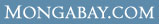# WHAT ARE THE AVERAGE Wages & salaries for Computer and mathematical occupations in Decatur, IL ?

 Overview Every year U.S. Department of Labor's Bureau of Labor Statistics releases employment and wage figures. This data was released in May 2006 and covers Wages & salaries for Computer and mathematical occupations in Decatur, IL Computer and mathematical occupations (Occupation [OCC] code: 15-0000) in Decatur, IL (BLS Area code: 19500 ) earned an average wage of \$27.85 per hour and an average annual salary of \$57,930 The BLS estimates that in Decatur, IL there were around 310 employed in the field of Computer and mathematical occupations.

Industry Comparison
Industry comparison
Computer and mathematical occupations in Decatur, IL earned 16% less than average for Computer and mathematical occupations in the United States.

Region/area/city comparison
Computer and mathematical occupations in Decatur, IL earned 67% more than the average worker in Decatur, IL.

State salary comparison
Computer and mathematical occupations in Decatur, IL earned 32% more than the average worker in Illinois.

National salary comparison
Computer and mathematical occupations in Decatur, IL earned 48% more than the average worker in the United States.

Wage and salary details
More detailed employment information for Computer and mathematical occupations in Decatur, IL

Hourly wage data for Computer and mathematical occupations in Decatur, IL

 Mean hourly wage: \$27.85 Tenth (10th) percentile hourly wage: \$13.40 Twenty-fifth (25th) percentile hourly wage: \$19.49 Fiftieth (50th) percentile wage [median] hourly wage: \$26.44 Seventy-fifth (75th) percentile hourly wage: \$34.89 Ninetieth (90th) percentile hourly wage: \$44.25

Salary figures for Computer and mathematical occupations in Decatur, IL

 Mean annual salary: \$57,930 Tenth (10th) percentile annual salary: \$27,870 Twenty-fifth (25th) percentile annual salary: \$40,530 Fiftieth (50th) percentile wage [median] annual salary: \$55,000 Seventy-fifth (75th) percentile annual salary: \$72,570 Ninetieth (90th) percentile annual salary: \$92,040

Indexwhat's new | rainforests home | help support the site | madagascar | search | about | contact